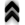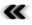### 7.2 Tracing Phase Space – Components from Theory

Where Section 7.1 described the dynamical system due to Boltzmann’s equation using diﬀerential equations, an approach using the integral form of Boltzmann’s equation as introduced in Section 5.6 is provided here. The presented integral equation is approached using the formalism described in Appendix A to approximate solutions. In contrast to the contraction to macroscopic quantities, as described in Section 7.1 this approach makes direct use of the phase space and its geometry by casting trajectories through it. As already outlined in Section 5.4, trajectories alone are no longer suﬃcient for an adequate description but a mechanism to change between trajectories is also required. The theory outlined in Section 5.6 is ﬁrst theoretically further reﬁned in order to provide guidelines usable for implementation, reusing components from Section 6.

The continuous model of the evolution of the dynamical system described in Section 5.6, needs only minor adaptations in order to be made accessible to the discrete world of digital computers. The adaptation is brieﬂy outlined to provide a guide of how independent components may be assembled to be applied to a dynamical system.

Since Boltzmann’s equation can be cast in the form of an integral equation, it is possible to construct a resolvent series. Recalling Equation 5.51b: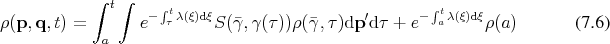Proceeding as outlined in Appendix A by recursive insertions leads to:It contains two scattering events, hence uses three diﬀerent trajectories (Deﬁnition 46), which are connected at their end points by the condition that they agree in their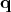component using the corresponding parameter, while allowing discontinuous transitions in their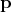components, as already established in Equation 5.47. Recalling it in adapted form it reads: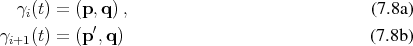Another important fact is that the sequence of trajectories here, actually moves back in time from the point of interest to the initial point. Thus the algorithms utilizing this approach directly are aptly called backward algorithms .

The trajectories encountered here correspond to the integral curves (Deﬁnition 63) of the Hamiltonian vector ﬁeld (Deﬁnition 62). Each trajectory thus is a representative of the Hamiltonian ﬂow (Deﬁnition 67), which governs the evolution of the system as a whole. Thus it becomes apparent that the solution is obtained by tracing the trajectories which follow the basic structures which deﬁne the geometry of the problem domain.

In the spirit of applying a Monte Carlo approach for the evaluation of the integrals, as described in Section 6.6.2 the factors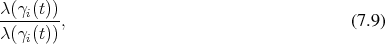which leave the value unchanged but allow for easier interpretation are introduced. After introduction of these factors and slight reordering the following is obtained: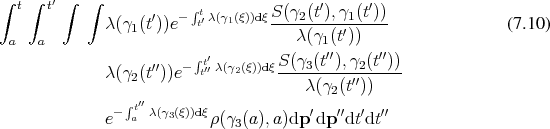This identiﬁes the terms of the form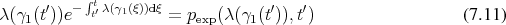as the probability distribution function corresponding to the exponential distribution (Deﬁnition 103). While it is highly tempting to also identify the terms of the form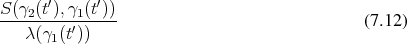with a probability distribution (Deﬁnition 99), it is not the case as the integration deﬁning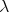is not carried out with the corresponding states. However, such a term can easily by introduced recalling Equation 5.43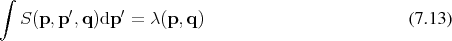and analogously setting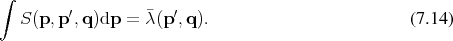Inserting this expression provides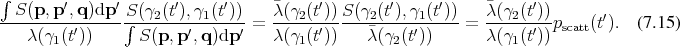Thus setting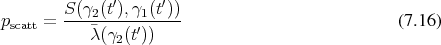corresponds to a random variable of uniform distribution (Deﬁnition 102). Application of this to Equation 7.10 yields: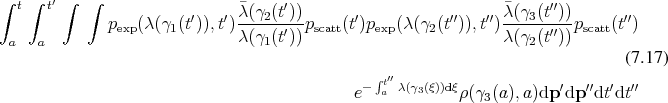The ﬁnal remaining exponential can be expressed as an additional integral over the parameter again representing an exponential distribution, thus ﬁnally obtaining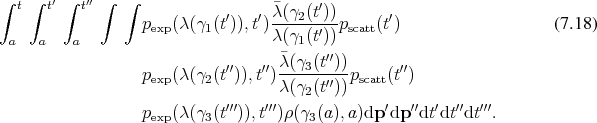While this expression is particular to the second term of the iteration, all following terms follow the same pattern and are expressible as a series of multiplications of probabilities along with a corresponding density.

The key components required for the approximation of the described dynamical system can be found from Equation 7.18 to be:

• Sections of trajectories moving through phase space
• Transitions from one trajectory to another
• Random number generation (see Appendix B)

#### 7.2.1 Sections of Trajectories

Trajectories have already been introduced in Section 6.5 under the name of curves which shall be specialized for the context of dynamics. While trajectories corresponding to a Hamiltonian ﬂow (Deﬁnition 67) ﬁbrate (Deﬁnition 39) the whole phase space, only sections are required for the treatment of the dynamical system described by Boltzmann’s equation.

Nevertheless, the sections of the trajectories under investigation are integral curves (Deﬁnition 63) of the Hamiltonian vector ﬁeld (Deﬁnition 62) deﬁned on the phase space. Considering the phase space as the co-tangent bundle leads to a split of this vector ﬁeld, into two components which are still tangential to the curve. However, the two separated components are no longer each a vector ﬁeld. Where the component corresponding to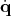may be immediately identiﬁed with a vector ﬁeld corresponding to the velocity, the part representing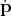is a tensor ﬁeld (Deﬁnition 65) of type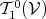, thus a ﬁeld of-forms, not a vector ﬁeld. This ﬁeld is given by the-forms corresponding to the force acting at each point of the manifold. While the nature of the components seems to have changed when projecting to the base manifold structures, both ﬁelds remain to be an expression of the Hamiltonian which deﬁned the symplectic geometry.

The extent of the section of the trajectory traversed corresponds to a random variable using the exponential distribution described in Equation 7.11. A random variable (Deﬁnition 98) with the desired properties can be generated from a uniformly distributed random variable by inversion of the distribution function as outlined in Appendix B. Recalling Equation 7.11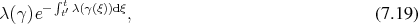the cumulative distribution function to this probability density distribution reads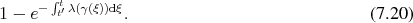Equating this expression with a uniformly distributed random variable, e.g., from the interval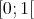, allows to formulate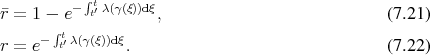The new variable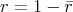is again a uniformly distributed random variable from the interval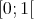. This expression can readily be inverted to yield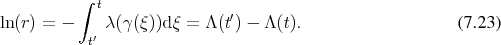The domain of interest and the quantities associated with it are represented in a discrete manner in the digital computer as described in Section 6.3 and Section 6.4. The discrete nature has repercussions on the validity of the considered trajectory, such that it models only the local ﬂow (Deﬁnition 66) instead of the original global ﬂow. The border of validity of any given local trajectory is due to the ﬁnite size of the subdivision into cells (Deﬁnition 34 and discussed in Section 6.3), which form the base space of the ﬁber bundle (Deﬁnition 40 as implemented in Section 6.4) carrying the quantities which determine the shape of the trajectories.

Since the trajectories are nothing else than curves through phase space, the shape of the the trajectories is completely deﬁned by the coeﬃcients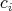, which were abstractly deﬁned in Section 6.5. Here, they can be associated to physical quantities.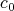corresponds to the position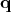of a particle, whileis linked to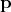. Finally,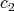represents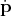. Thus the geometry of the trajectory is still tightly tied to the entities of the original symplectic structure.

The discrete nature makes it necessary to check, if the trajectory leaves the originating cell with any chosen value of the parameter and therefore actually serves as an upper limit for how far the trajectory is traversed, since the trajectory needs to be interrupted in case the parameter carries the new position beyond the initial cell, as already outlined in Section 6.5. Since the trajectories are one-dimensional, it is suﬃcient to compare the parameters corresponding to the intersection of a trajectory with a current cell’s boundary to the value of the parameter obtained from the random distribution.

#### 7.2.2 Trajectory Hopping

Both descriptions of Boltzmann’s equation as provided in Section 4.9 and Section 5.6 acknowledge the deﬁning nature of the scattering term on the right hand side. Thus the scattering term has to be mapped adequately to the realm of digital computing in order to expect the calculated result to be accurate. Furthermore, the scattering term is, together with the geometry deﬁning Hamiltonian, the point of entry for physical modelling into the mathematical structure. Thus, the inclusion of diﬀerent scattering mechanisms needs not only to be adequate from a mathematical point of view but also must withstand a physicist’s scrutiny.

The scattering kernel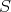is therefore modelled as being composed from diﬀerent scattering mechanisms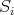such that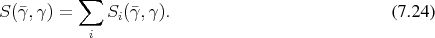Thus, recalling Equation 7.14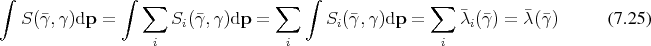and by Equation 7.16, which reads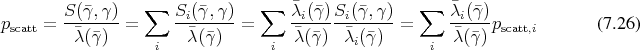the probabilities for each of the individual scattering mechanisms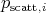can be related to the total scattering probability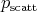. This relation also allows the selection of a given scattering mechanism by generating a uniformly distributed random variable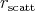from the interval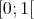. Since it is possible to uniquely identify the contribution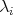of every scattering mechanism by its index, it is possible to select a scattering mechanism by choosing an index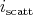. When choosing this index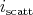, the following inequality holds.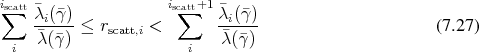Equivalently, it is possible to forgo normalization for the selection, since it is to a common factor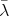and expressing it in terms of rates as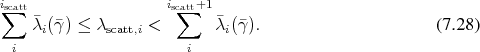The nature of continued summation determines the scattering probability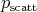along with the selection of the scattering mechanism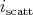, which determines the beginning of the new trajectory, by means of the rejection technique as is outlined in Appendix B.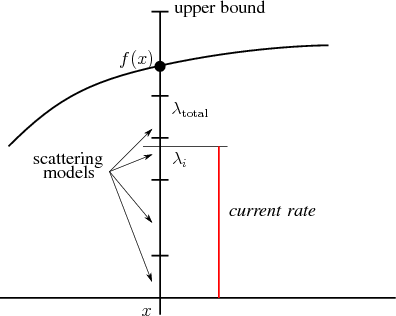Figure 7.1: Schematic illustration of the rejection technique to evaluate scattering mechanisms.

Figure 7.1 provides an illustration of this particular case. At a given point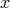a current rate is chosen randomly using a distribution from the interval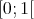multiplied by an upper bound for all the scattering. Then the scattering models are evaluated at this point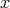and the individual rates, thusly calculated are summed in order to determine the interval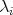, which contains the current rate. The scattering mechanism associated with this interval is then chosen as the new state from which the next trajectory will propagate.

During the simulation the scattering models are evaluated repeatedly in order to choose the next state of the electron under consideration. Therefore high performance is among the requirements placed on the evaluation of the collision model.

#### 7.2.3 Bridging Two Worlds

Applications implementing scattering mechanisms encountered in the ﬁeld of solid state electronics, which change the states of electrons have been available quite some time. The foundations for the presented code have been been written in plain ANSI C, where all available scattering mechanisms are implemented as individual functions, which are called subsequently. The scattering models require a variable set of parameters, which leads to non-homogeneous interfaces in the functions representing them. To alleviate this to some extent global variables have been employed completely eliminating any aspirations of data encapsulation and posing a serious problem for attempts for parallelization to take advantage of multi-core CPUs. The code has the very simple and repetitive structure:

double sum = 0;
double current_rate = generate_random_number();

if (A_key == on)
{
sum = A_rate(state, parameters);

if (current_rate < sum)
{
counter>A[state>valley]++;
state_after_A (st, p);
return
}
}
if (B_key == on)
{     ...    }
...

Extensions to this code are usually accomplished by copy and paste, which is prone to simple mistakes by oversight, such as failing to change the counter which has to be incremented or calling the incorrect function to update the electron’s state.

Furthermore, at times the need arises to calculate the sum of all the scattering models (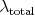in Figure 7.1), which is accomplished in a diﬀerent part of the implementation, thus further opening the possibility for inconsistencies between the two code paths.

The decision which models to evaluate is performed strictly at run time and it would require signiﬁcant, if simple, modiﬁcation of the code to change this at compile time, thus making highly optimized specializations very cumbersome.

The functions calculating the rates and state transitions, however, have been well tested and veriﬁed, so that abandoning them would be wasteful.

Object oriented programming directly oﬀers run time polymorphism by means of virtual inheritance. Unfortunately current implementations of inheritance use an intrusive approach for new software components and tightly couple a type and the corresponding operations to the super type. In contrast to object-oriented programming, current applications of generic programming are limited to algorithms using statically and homogeneously typed containers, but oﬀer highly ﬂexible, reusable, and optimizeable software components.

As can be seen, both programming types oﬀer diﬀerent points of evaluation. Run time polymorphism based on run time concepts  tries to combine the virtual inheritance run time modiﬁcation mechanism with the compile time ﬂexibility and optimization.

Inheritance in the context of run time polymorphism is used to provide an interface template to model the required concept, where the derived class must provide the implementation of the given interface. The following code snippet

{
typedef StateT state_type;
boost::shared_ptr<scattering_concept> scattering_object;

struct scattering_concept
{
virtual scattering_concept() {} ;
virtual numeric_type rate(const state_type& input) const = 0;
virtual void transition(state_type& input) = 0;
}

template<typename T> struct scattering_model :
scattering_concept
{
T scattering_instance;
scattering_model(const T& x) : scattering_instance(x) {}
numeric_type rate(const state_type& input) const ;
void transition(state_type& input) ;
}

numeric_type rate(const state_type& input) const
void transition(state_type& input) ;
template<typename T>
scattering_object(new scattering_model<T>(x)) {}
};

therefore introduces a scattering_facade which wraps a scattering_concept part. The virtual inheritance is used to conﬁgure the necessary interface parts, in this case rate() and transition(), which have to be implemented by any scattering model. In the given example the state_type is still available for explicit parametrization.

To interface this novel approach a core structure is implemented which wraps the implementations of the scattering models:

template<typename ParameterType>
struct scattering_rate_A
{
...
const ParameterType& parameters;

scattering_rate_A (const ParameterType& parameters):
parameters(parameters) {}

template <typename StateType> numeric_type
operator() (const StateType& state) const
{
return A_rate(state, parameters);
}
};

By supplying the required parameters at construction time it is possible to homogenize the interface of the operator(). This methodology also allows the continued use of the old data structures in the initial phases of transition, while not being so constrictive as to hamper future developments.

The functions for the state transitions are treated similarly to those for the rate calculation. Both are then fused in a scattering_pack to form the complete scattering model and to ensure consistency of the rate and state transition calculations as can be seen in the following part of code:

template<scattering_rate_type, transition_type, parameter_type>
struct scattering_pack
{
scattering_rate_type<parameter_type>  rate_calculation;
transition_type<parameter_type>  state_transition;

scattering_pack  (const parameter_type& parameters) :
rate_calculation(parameters), state_transition(parameters)
{}

template<typename StateType>
numeric_type rate(const StateType& state) const
{
return rate_calculation(state);
}

template<typename StateType>
void transition(StateType& state)
{
state_transition(state);
}
}

The blend of run time and compile time mechanisms allows the storage of all scattering models within a single container, e.g. std::vector, which can be iterated over in order to evaluate them.

scatter_container_type ;

scatter_container_type scatter_container ;
scatter_container.push_back(scattering_model) ;

For the development of new collision models easy extendability, even without recompilations, is also a highly important issue. This approach allows the addition of scattering models at run time and to expose an interface to an interpreted language such as, e.g., Python .

In case a highly optimized version is desired, the run time container (here the std::vector) may be exchanged by a compile time container, which is also readily available from the GSSE and provides the compiler with further opportunities for optimizations at the expense of run time adaptability.

While the described approach initially slightly increases the burden of implementation, due to the fact that wrappers must be provided, it gives a transition path to integrate legacy codes into an up to date frame while at the same time not abandoning the experience associated with it. The invested eﬀort allows to raise the level of abstraction, which in turn allows to increase the beneﬁts obtained from the advances in compiler technologies. This in turn inherently allows an optimization for several platforms without the need for massive human eﬀort, which was needed in previous approaches.

In this particular case, encapsulating the reliance on global variables of the functions implementing the scattering models to the wrapping structures, parallelization eﬀorts are greatly facilitated, which are increasingly important with the continued increase of computing cores per CPU.

Furthermore the results can easily be veriﬁed as code parts are gradually moved to newer implementations, the only stringent requirement being link compatibility with C++. This test and veriﬁcation can be taken a step further in case the original implementation is written in ANSI C, due to the high compatibility of it to C++. It is possible to weave parts of the new implementation into the older code. Providing the opportunity to get a comparison not only of ﬁnal results, but of all the intermediates as well.

Such swift veriﬁcation of implementations allows to also speed up the steps necessary to verify calculated results with subsequent or contemporary experiments, which should not be neglected, in order to keep physical models and their numerical representations strongly rooted in reality.

The correct operation of the run time concepts depends on the faultless operation of the compiler being used within the rules set down by the C++ language speciﬁcation . Under this crucial assumption the correct compilation of the code ensures that the code will behave according to the guarantees introduced by the run time concept. While this provides an indication for correctness it cannot be considered absolute proof, especially since the C++ language and its compilers does not have a special focus on validation as is the case in, e.g., Ada, which could certainly make a stronger case.